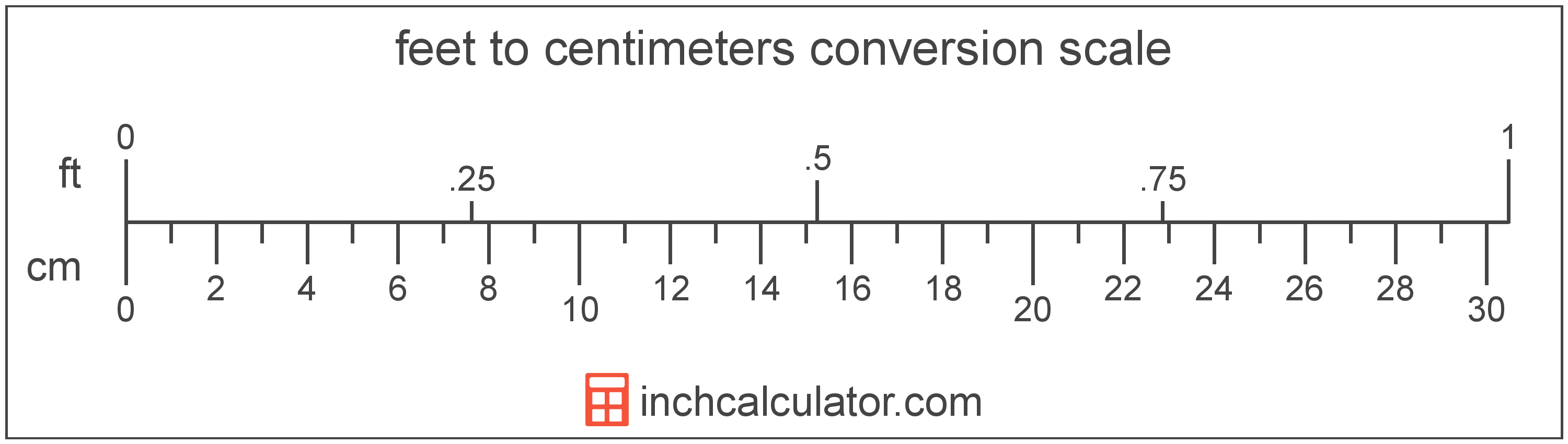# Centimeters to Feet & Inches Conversion

Enter the length in centimeters below to get the value converted to feet.

Results in Feet:1 cm = 0.032808 ft
1 cm = 0' 25/64"
Do you want to convert feet to centimeters?

## How to Convert Centimeters to FeetTo convert a centimeter measurement to a foot measurement, multiply the length by the conversion ratio.

Since one centimeter is equal to 0.032808 feet, you can use this simple formula to convert:

feet = centimeters × 0.032808

The length in feet is equal to the centimeters multiplied by 0.032808.

For example, here's how to convert 5 centimeters to feet using the formula above.
5 cm = (5 × 0.032808) = 0.164042'

Our inch fraction calculator can add centimeters and feet together, and it also automatically converts the results to US customary, imperial, and SI metric values.

## Centimeters

One centimeter is equal to one-hundredth (1/100) of a meter, which is defined as the distance light travels in a vacuum in a 1/299,792,458 second time interval.

The centimeter, or centimetre, is a multiple of the meter, which is the SI base unit for length. In the metric system, "centi" is the prefix for 10-2. Centimeters can be abbreviated as cm; for example, 1 centimeter can be written as 1 cm.

Metric rulers typically have 30 cm, which are represented by 30 large tick marks. To get a rough idea of the actual length of a centimeter, a standard pencil is just about 1 cm thick.

## Feet

The foot is a unit of linear length measure equal to 12 inches or 1/3 of a yard. Because the international yard is legally defined to be equal to exactly 0.9144 meters, one foot is equal to 0.3048 meters.

The foot is a US customary and imperial unit of length. Feet can be abbreviated as ft; for example, 1 foot can be written as 1 ft.

Feet can also be denoted using the symbol, otherwise known as a prime, though a single-quote (') is often used instead of the prime symbol for convenience. Using the prime symbol, 1 ft can be written as 1′.

The foot is most commonly measured using either a standard 12" ruler or a tape measure, though there are many other measuring devices available. Feet are sometimes referred to as linear feet, which are simply the measurement of length in feet.

You might be interested in our feet and inches calculator, which can add feet with inches, centimeters, or meters.

We recommend using a ruler or tape measure for measuring length, which can be found at a local retailer or home center. Rulers are available in imperial, metric, or combination with both values, so make sure you get the correct type for your needs.

Need a ruler? Try our free downloadable and printable rulers, which include both imperial and metric measurements.

## Centimeter to Foot Conversion Table

Centimeter measurements converted to feet
Centimeters Feet
1 cm 0.032808'
2 cm 0.065617'
3 cm 0.098425'
4 cm 0.131234'
5 cm 0.164042'
6 cm 0.19685'
7 cm 0.229659'
8 cm 0.262467'
9 cm 0.295276'
10 cm 0.328084'
11 cm 0.360892'
12 cm 0.393701'
13 cm 0.426509'
14 cm 0.459318'
15 cm 0.492126'
16 cm 0.524934'
17 cm 0.557743'
18 cm 0.590551'
19 cm 0.62336'
20 cm 0.656168'
21 cm 0.688976'
22 cm 0.721785'
23 cm 0.754593'
24 cm 0.787402'
25 cm 0.82021'
26 cm 0.853018'
27 cm 0.885827'
28 cm 0.918635'
29 cm 0.951444'
30 cm 0.984252'
31 cm 1.0171'
32 cm 1.0499'
33 cm 1.0827'
34 cm 1.1155'
35 cm 1.1483'
36 cm 1.1811'
37 cm 1.2139'
38 cm 1.2467'
39 cm 1.2795'
40 cm 1.3123'

## References

1. National Institute of Standards and Technology, U.S. Survey Foot: Revised Unit Conversion Factors, https://www.nist.gov/pml/us-surveyfoot/revised-unit-conversion-factors